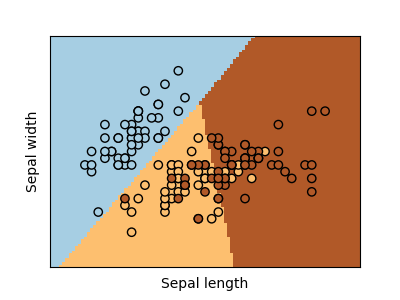# Logistic Regression 3-class Classifier¶

Show below is a logistic-regression classifiers decision boundaries on the first two dimensions (sepal length and width) of the iris dataset. The datapoints are colored according to their labels.# Code source: Gaël Varoquaux
# Modified for documentation by Jaques Grobler

import matplotlib.pyplot as plt
from sklearn.linear_model import LogisticRegression
from sklearn import datasets
from sklearn.inspection import DecisionBoundaryDisplay

# import some data to play with
X = iris.data[:, :2]  # we only take the first two features.
Y = iris.target

# Create an instance of Logistic Regression Classifier and fit the data.
logreg = LogisticRegression(C=1e5)
logreg.fit(X, Y)

_, ax = plt.subplots(figsize=(4, 3))
DecisionBoundaryDisplay.from_estimator(
logreg,
X,
cmap=plt.cm.Paired,
ax=ax,
response_method="predict",
plot_method="pcolormesh",
xlabel="Sepal length",
ylabel="Sepal width",
eps=0.5,
)

# Plot also the training points
plt.scatter(X[:, 0], X[:, 1], c=Y, edgecolors="k", cmap=plt.cm.Paired)

plt.xticks(())
plt.yticks(())

plt.show()


Total running time of the script: ( 0 minutes 0.065 seconds)

Gallery generated by Sphinx-Gallery